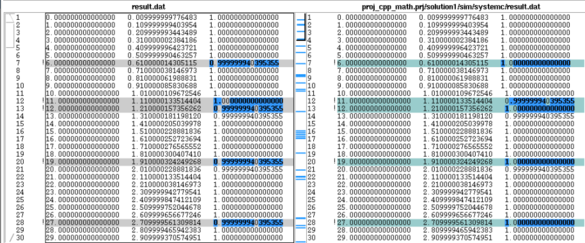# Verification Option 1: Standard Math Library and Verify Differences - 2022.1 English

## Vitis High-Level Synthesis User Guide (UG1399)

Document ID
UG1399
Release Date
2022-06-07
Version
2022.1 English

In this option, the standard C math libraries are used in the source code. If any of the functions synthesized do have exact accuracy the C/RTL co-simulation is different than the C simulation. The following example highlights this approach.

``````#include <cmath>
#include <fstream>
#include <iostream>
#include <iomanip>
#include <cstdlib>
using namespace std;

typedef float data_t;

data_t cpp_math(data_t angle) {
data_t s = sinf(angle);
data_t c = cosf(angle);
return sqrtf(s*s+c*c);
}``````

In this case, the results between C simulation and C/RTL co-simulation are different. Keep in mind when comparing the outputs of simulation, any results written from the test bench are written to the working directory where the simulation executes:

• C simulation: Folder <project>/<solution>/csim/build
• C/RTL co-simulation: Folder <project>/<solution>/sim/<RTL>

where `<project>` is the project folder, `<solution>` is the name of the solution folder and `<RTL>` is the type of RTL verified (Verilog or VHDL). The following figure shows a typical comparison of the pre-synthesis results file on the left-hand side and the post-synthesis RTL results file on the right-hand side. The output is shown in the third column.

Figure 1. Pre-Synthesis and Post-Synthesis Simulation DifferencesThe results of pre-synthesis simulation and post-synthesis simulation differ by fractional amounts. You must decide whether these fractional amounts are acceptable in the final RTL implementation.

The recommended flow for handling these differences is using a test bench that checks the results to ensure that they lie within an acceptable error range. This can be accomplished by creating two versions of the same function, one for synthesis and one as a reference version. In this example, only function `cpp_math` is synthesized.

``````#include <cmath>
#include <fstream>
#include <iostream>
#include <iomanip>
#include <cstdlib>
using namespace std;

typedef float data_t;

data_t cpp_math(data_t angle) {
data_t s = sinf(angle);
data_t c = cosf(angle);
return sqrtf(s*s+c*c);
}

data_t cpp_math_sw(data_t angle) {
data_t s = sinf(angle);
data_t c = cosf(angle);
return sqrtf(s*s+c*c);
}
``````

The test bench to verify the design compares the outputs of both functions to determine the difference, using variable `diff` in the following example. During C simulation both functions produce identical outputs. During C/RTL co-simulation function `cpp_math` produces different results and the difference in results are checked.

``````int main() {
data_t angle = 0.01;
data_t output, exp_output, diff;
int retval=0;

for (data_t i = 0; i <= 250; i++) {
output = cpp_math(angle);
exp_output = cpp_math_sw(angle);

// Check for differences
diff = ( (exp_output > output) ? exp_output - output : output - exp_output);
if (diff > 0.0000005) {
printf("Difference %.10f exceeds tolerance at angle %.10f \n", diff, angle);
retval=1;
}

angle = angle + .1;
}

if (retval != 0) {
printf("Test failed  !!!\n");
retval=1;
} else {
printf("Test passed !\n");
}
// Return 0 if the test passes
return retval;
}
``````

If the margin of difference is lowered to 0.00000005, this test bench highlights the margin of error during C/RTL co-simulation:

``````Difference 0.0000000596 at angle 1.1100001335
Difference 0.0000000596 at angle 1.2100001574
Difference 0.0000000596 at angle 1.5100002289
Difference 0.0000000596 at angle 1.6100002527
etc..
``````

When using the standard C math libraries (math.h and cmath.h) create a “smart” test bench to verify any differences in accuracy are acceptable.